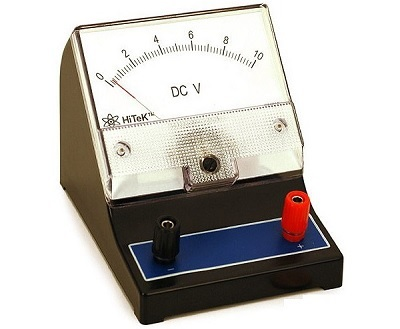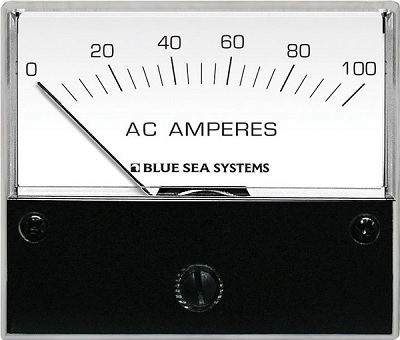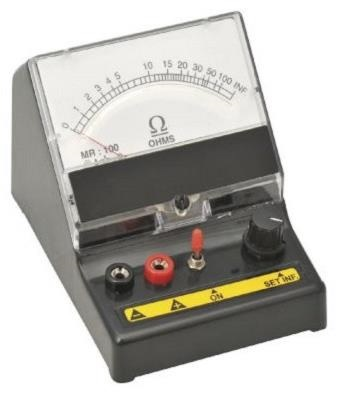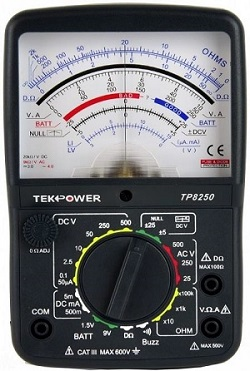# Introduction

The instruments, which are used to measure any quantity are known as measuring instruments. This tutorial covers mainly the electronic instruments, which are useful for measuring either electrical quantities or parameters.

Following are the most commonly used electronic instruments.

• Voltmeter
• Ammeter
• Ohmmeter
• Multimeter

Now, let us discuss about these instruments briefly.

## Voltmeter

As the name suggests, voltmeter is a measuring instrument which measures the voltage across any two points of an electric circuit. There are two types of voltmeters: DC voltmeter, and AC voltmeter.

DC voltmeter measures the DC voltage across any two points of an electric circuit, whereas AC voltmeter measures the AC voltage across any two points of an electric circuit. An example of practical DC voltmeter is shown in below figure.The DC voltmeter shown in above figure is a $(0-100)V$ DC voltmeter. Hence, it can be used to measure the DC voltages from zero volts to 10 volts.

## Ammeter

As the name suggests, ammeter is a measuring instrument which measures the current flowing through any two points of an electric circuit. There are two types of ammeters: DC ammeter, and AC ammeter.

DC ammeter measures the DC current that flows through any two points of an electric circuit. Whereas, AC ammeter measures the AC current that flows through any two points of an electric circuit. An example of practical AC ammeter is shown in below figure −The AC ammeter shown in above figure is a $(0-100)A \:$ AC ammeter. Hence, it can be used to measure the AC currents from zero Amperes to 100 Amperes.

## Ohmmeter

Ohmmeter is used to measure the value of resistance between any two points of an electric circuit. It can also be used for finding the value of an unknown resistor. There are two types of ohmmeters: series ohmmeter, and shunt ohmmeter.

In series type ohmmeter, the resistor whose value is unknown and to be measured should be connected in series with the ohmmeter. It is useful for measuring high values of resistances.In shunt type ohmmeter, the resistor whose value is unknown and to be measured should be connected in parallel (shunt) with the ohmmeter. It is useful for measuring low values of resistances.

An example of practical shunt ohmmeter is shown in the above figure. The ohmmeter shown in above figure is a $(0-100)\Omega$ shunt ohmmeter. Hence, it can be used to measure the resistance values from zero ohms to 100 ohms.

## Multimeter

Multimeter is an electronic instrument used to measure the quantities such as voltage, current & resistance one at a time. It can be used to measure DC & AC voltages, DC & AC currents and resistances of several ranges. A practical multimeter is shown in the following figure −As shown in the figure, this multimeter can be used to measure various high resistances, low resistances, DC voltages, AC voltages, DC currents, & AC currents. Different scales and range of values for each of these quantities are marked in above figure.

The instruments which we considered in this chapter are of indicating type instruments, as the pointers of these instruments deflect and point to a particular value. We will discuss about these electronic measuring instruments in detail in later chapters.